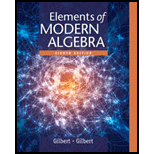# Decide whether the given set B is closed with respect to the binary operation defined on the set of integers Z . If B is not closed, exhibit elements x ∈ B and y ∈ B , such that x ∗ y ∉ B . a. x ∗ y = x y , B = { − 1 , − 2 , − 3 , … } b. x ∗ y = x − y , B = Z + c. x ∗ y = x 2 + y 2 , B = Z + d. x ∗ y = sgn x + sgn y , B = { − 2 , − 1 , 0 , 1 , 2 } where sgn x = { 1 if x &gt; 0 0 if x = 0 − 1 if x &lt; 0 e. x ∗ y = | x | − | y | , B = Z + f. x ∗ y = x + x y , B = Z + g. x ∗ y = x y − x − y , B is the set of all odd integers. h. x ∗ y = x y , B is the set of positive odd integers.### Elements Of Modern Algebra

8th Edition
Gilbert + 2 others
Publisher: Cengage Learning,
ISBN: 9781285463230### Elements Of Modern Algebra

8th Edition
Gilbert + 2 others
Publisher: Cengage Learning,
ISBN: 9781285463230

#### Solutions

Chapter
Section
Chapter 1.4, Problem 1E
Textbook Problem

## Expert Solution

### Want to see the full answer?

Check out a sample textbook solution.See solution

### Want to see this answer and more?

Experts are waiting 24/7 to provide step-by-step solutions in as fast as 30 minutes!*

See Solution

*Response times vary by subject and question complexity. Median response time is 34 minutes and may be longer for new subjects.